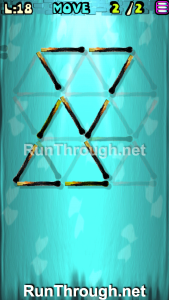# Move the Matches Puzzles Episode 3 Level 1-20 Walkthrough

Walkthrough for all the solutions and answers to the game Move the Matches Puzzles by K-Lab. Get the app on the Google Play Store to play along if you do not already have it.  This is for episode 3, levels 1 through 20.

The images show the final state of the puzzle, so you have to add, move, or remove matches to get the exact picture shown here in this walkthrough.

Enjoy this walkthrough, and let us know what other games you want us to have a walkthrough or review for!

### Level 1Instructions: Move 4 matches to get 3 triangles

###Instructions: Remove 4 matches to get 5 squares

###Instructions: Remove 2 matches to correct the equation

Solution: 5-0=5

###Instructions: Move 2 matches to get 3 triangles, all equal

###Instructions: Move 2 matches to correct the equation

Solution: 9-6=3

###Instructions: Remove 2 matches to correct the equation

Solution: 3+8=11

###Instructions: Add 3 matches to correct the equation

Solution: 7-4=3

###Instructions: Move 2 matches to get 5 triangles

###Instructions: Move 2 matches to correct the equation

Solution: 7×9=63

###Instructions: Remove 2 matches to correct the equation

Solution: 9/9=1

###Instructions: Remove 7 matches to get 3 triangles

###Instructions: Add 1 match to correct the equation

Solution: 8+5=13

###Instructions: Add 2 matches to correct the equation

Solution: 4×6=24

###Instructions: Move 2 matches to correct the equation

Solution: 2×2=4

###Instructions: Add 2 matches to get 3 triangles

###Instructions: Remove 3 matches to correct the equation

Solution: 2/1=2

###Instructions: Move 2 matches to correct the equation

Solution: 3×3=9

###Instructions: Move 2 matches to get 2 triangles

###Instructions: Move 2 matches to correct the equation

Solution: 5+9=14

### Level 20Instructions: Add 1 match to correct the equation

Solution: 8×5=40